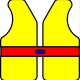# Converting metres & centimetres, length, area and volume

Many people incorrectly assume that as there are 100 cm in a metre then there must also be 100 cm2 in 1 m2 and 100 cm3 and 1 m3. Working through the pictures below should convince you that there are:

100 cm in 1 m but
10,000 cm2 in 1 m2
and 1,000,000 cm3 in 1 m3

### Length1 m = 100 cm### Area1 m2    = 100 × 100 = 10 000 cm2

= 104 cm2### Volume1 m3    =  100 × 100 × 100 = 1 000 000 cm3

= 106 cm3one litre = 1000 cm3

1 l = 1000 cm3

1 m3 = 1000 l

Now read this post in conjunction with the one on units for area and volume! The units for area are square metres and the units for volume are cubic metres. Don’t confuse either of these with metres square or metres cubed!Posted on Categories Maths

This site uses Akismet to reduce spam. Learn how your comment data is processed.